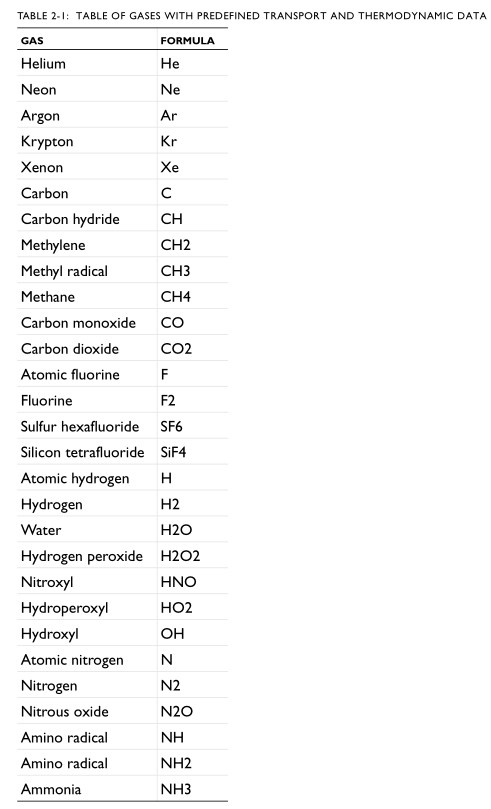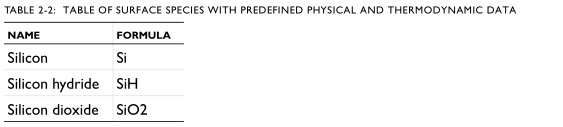# Comsol等离子体模块使用手册

## 2. 等离子体模型所需数据

### 2.1 所需数据

#### 2.1.1 电子撞击反应

https://fr.lxcat.net/home/

• 用于弹性碰撞的电子与目标物质的质量比。
• 非弹性碰撞的能量损失（以 eV 为单位）。
• 如果需要详细的平衡，目标和生产物种之间的统计权重比。

DETAILED BALANCE细节平衡？

$e+Ar=>e+Ars$

$e+Ars=>e+Ar$

#### 2.1.3 界面反应

• 正向粘附或速率系数，可以是数值或根据阿累尼乌斯参数指定为表面温度的函数。
• 对于一级反应，需要总表面位点浓度。
• 对于导致发射二次电子的表面反应，需要二次发射系数和二次电子的平均能量。

#### 2.1.4 类

• 物种的分子量
• 物种的潜在特征长度； 需要计算正确的扩散率和迁移率
• 物种的势能最小值； 需要计算正确的扩散率和迁移率

• 物种摩尔焓、熵和比热，可以直接作为温度的函数或使用 NASA 多项式输入
• 在电子激发的物质或离子的情况下，可以输入基态物质的性质，也可以输入对物质焓的额外贡献• Thermodynamic properties: http://burcat.technion.ac.il/dir/THERM.DAT • Transport properties: http://uigelz.eecs.umich.edu/pub/data/e_reactions.pdf • Additional transport properties: http://combustion.berkeley.edu/gri-mech/ version30/files30/transport.dat

### 2.2 导入碰撞横截面数据

• 横截面数据文件格式
• 文件格式
• 等离子模块横截面数据要求的参考资料

## 3. AC/DC 接口

• 静电接口
• 电流接口
• 电路接口
• 静电接口理论
• 电流接口理论
• 电路接口理论
• 连接到电路
• 材料导入

### 3.1 静电接口

#### 3.1.1 静电接口的域、边界、边、点和对节点

$\mathbf{n}{2} \cdot\left(\mathbf{D}{1}-\mathbf{D}{2}\right)=\rho{\mathrm{s}}$

$\mathbf{n} \cdot\left[\left(\varepsilon_{0} \nabla V-\mathbf{P}\right){1}-\left(\varepsilon{0} \nabla V-\mathbf{P}\right){2}\right]=-\mathbf{n} \cdot\left(\mathbf{D}{1}-\mathbf{D}_{2}\right)=0$